Voltage Drop Calculation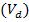For single phase circuits: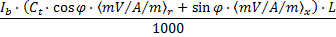For three phase circuits:With: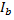the design current of the circuit under consideration in Amperesthe tabulated resistance/reactance of the conductor taken from the tables 4D1B–4J4B of BS 7671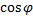the power factor of the load of the circuit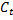the temperature correction factor (according to BS 7671 Appendix 4)the circuit length in meters

Note: For unbalanced 3 phase circuits, current will be flowing in the neutral conductor as illustrated in the phasor diagram below. The voltage drop in each phase is calculated by summing of the vectors of the phase and the neutral voltage drops.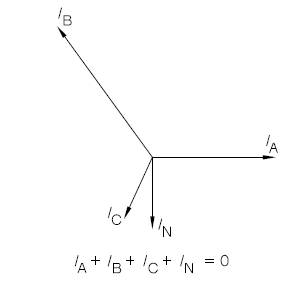Voltage Drop Limits Check

The software checks that the total voltage drop (between the origin of the installation and the circuit) is in the voltage drop limit set.

The voltage drop limits can be user defined or according to Table 4Ab in Appendix 4 of BS7671:2008 + A3:2015.